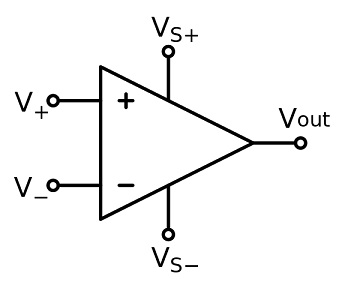# Difference Between Differential Amplifier and Operational Amplifier

## Main Difference – Differential Amplifier vs. Operational Amplifier

Amplifiers are extremely vital components in electronic circuits. The main difference between differential amplifier and operational amplifier is that a differential amplifier is an amplifier that amplifies a voltage difference between its inputs, whereas an operational amplifier is, in fact, a type of differential amplifier with a large open-loop gain, a high input impedance and a low output impedance.

## What is a Differential Amplifier

A differential amplifier is an electric component which amplifies the difference between two signals applied to two input terminals, while rejecting signals which are common to both the input terminals. A differential amplifier has two inputs: we will refer to these as$V_1$ and$V_2$. Suppose the amplifier amplifies$V_1$ by a factor$A_1$ and$V_2$ by a factor$A_2$. Then, the output voltage$V_o$ would be:$V_o=A_1V_1+A_2V_2\qquad(1)$.

The differential voltage ($V_d$) is defined as$V_d= V_2-V_1\qquad(2)$

The common mode voltage ($V_c$) is defined as$V_c=\frac{ V_1+V_2}{2}\Rightarrow 2V_c=V_1+V_2\qquad(3)$.

Using these two values, we can try to derive an expression for the output voltage.$(2)+(3)\Rightarrow 2V_c+V_d=2V_2\Rightarrow V_2=V_c+\frac{V_d}{2}$ and,$(2)-(3)\Rightarrow V_d+2V_c=-2V_1\Rightarrow V_1=V_c-\frac{V_d}{2}$

Now we can substitute these values back into$(1)$, giving us:$V_o=A_1\left( V_c-\frac{V_d}{2}\right)+A_2\left( V_c+\frac{V_d}{2}\right)$

Expanding this and re-factorizing, we get:$V_o=\left( A_1+A_2\right)V_c+\left( \frac{A_2-A_1}{2}\right)V_d$

The quantity$A_1+A_2$ is called the common-mode gain ($A_c$) and the quantity$\frac{A_2-A_1}{2}$ is called the differential gain ($A_d$). In terms of these nquantitietwo quantities, we can simply write the above equation as:$V_o=A_cV_c+A_dV_d$

## What is an Operational Amplifier

An operational amplifier is a type of differential amplifier with high gain. Operational amplifiers have large input impedances and small output impedances. For ideal operational amplifiers, the input impedance is taken to be infinite while the output impedance is taken to be 0. Often, operational amplifiers make use of a resistor to provide itself negative feedback. By changing the value of the resistor, it is possible to change the gain of an operational amplifier. Note that adding a resistor reduces the overall gain of an operational amplifier. When we calculated the gain in the previous section, we did so by assuming there is no feedback. Therefore, the gain we have calculated is the maximum possible gain for an amplifier, and it is called the open-loop gain since there is no feedback mechanism in the system.

Operational amplifiers have an extremely important place in analog computing where they are used to not only amplify signals but to invert voltages, add voltages, and to carry out differentiation and integration.

The figure below shows the symbol for an operational amplifier:The symbol of an operational amplifier$V_+$ and$V_-$ are are the input terminals, while$V_{S+}$ and$V_{S-}$ are the terminals to which a power supply is connected.$V_{out}$ is the output terminal.

The image below shows an operational amplifier:An operational amplifier

## Difference Between Differential Amplifier and Operational Amplifier

differential amplifier is a type of amplifier which amplifies a voltage difference between two of its inputs.

An operational amplifier is a type of differential amplifier with a large open-loop gain, a very high input impedance and a very low output impedance.

Image Courtesy:

“Op-amp pinouts.” by User:Omegatron (Own work) [CC BY-SA 3.0], via Wikimedia Commons

“Opamp” by lambda’s (Own work) [CC BY 2.0], via flickr